# getLoopTransfer

Open-loop transfer function at specified point using slLinearizer or slTuner interface

## Syntax

linsys = getLoopTransfer(s,pt)
linsys = getLoopTransfer(s,pt,sign)
linsys = getLoopTransfer(s,pt,temp_opening)
linsys = getLoopTransfer(s,pt,temp_opening,sign)
linsys = getLoopTransfer(___,mdl_index)
[linsys,info] = getLoopTransfer(___)

## Description

example

linsys = getLoopTransfer(s,pt) returns the point-to-point open-loop transfer function at the specified analysis point for the model associated with the slLinearizer or slTuner interface, s.The software enforces all the permanent loop openings specified for s when it calculates linsys. If you configured either s.Parameters, or s.OperatingPoints, or both, getLoopTransfer performs multiple linearizations and returns an array of loop transfer functions.

example

linsys = getLoopTransfer(s,pt,sign) specifies the feedback sign for computing the open-loop response. By default, linsys is the positive-feedback open-loop transfer function. Set sign to -1 to compute the negative-feedback open-loop transfer function for applications that assume the negative-feedback definition of linsys. Many classical design and analysis techniques, such as the Nyquist or root locus design techniques, use the negative-feedback convention.The closed-loop sensitivity at pt is equal to feedback(1,linsys,sign).

example

linsys = getLoopTransfer(s,pt,temp_opening) considers additional, temporary, openings at the point specified by temp_opening. Use an opening, for example, to calculate the loop transfer function of an inner loop, measured at the plant input, with the outer loop open.
linsys = getLoopTransfer(s,pt,temp_opening,sign) specifies temporary openings and the feedback sign.

example

linsys = getLoopTransfer(___,mdl_index) returns a subset of the batch linearization results. mdl_index specifies the index of the linearizations of interest, in addition to any of the input arguments in previous syntaxes. Use this syntax for efficient linearization, when you want to obtain the loop transfer function for only a subset of the batch linearization results.

example

[linsys,info] = getLoopTransfer(___) returns additional linearization information.

## Examples

collapse all

Obtain the loop transfer function, calculated at e, for the ex_scd_simple_fdbk model.

Open the ex_scd_simple_fdbk model.

mdl = 'ex_scd_simple_fdbk'; open_system(mdl);In this model:Create an slLinearizer interface for the model.

sllin = slLinearizer(mdl); 

To obtain the loop transfer function at e, add this point to sllin as an analysis point.

addPoint(sllin,'e'); 

Obtain the loop transfer function at e.

sys = getLoopTransfer(sllin,'e'); tf(sys) 
ans = From input "e" to output "e": -3 ----- s + 5 Continuous-time transfer function. 

The software adds a linearization output, breaks the loop, and adds a linearization input, de, at e.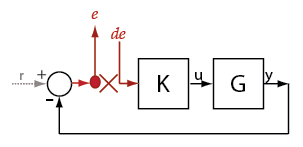sys is the transfer function from de to e. Because the software assumes positive-feedback, it returns sys as.

Obtain the negative-feedback loop transfer function, calculated at e, for the ex_scd_simple_fdbk model.

Open the ex_scd_simple_fdbk model.

mdl = 'ex_scd_simple_fdbk'; open_system(mdl);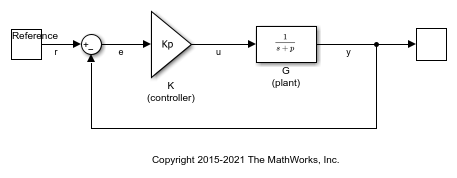In this model:Create an slLinearizer interface for the model.

sllin = slLinearizer(mdl); 

To obtain the loop transfer function at e, add this point to sllin as an analysis point.

addPoint(sllin,'e'); 

Obtain the loop transfer function at e.

sys = getLoopTransfer(sllin,'e',-1); tf(sys) 
ans = From input "e" to output "e": 3 ----- s + 5 Continuous-time transfer function. 

The software adds a linearization output, breaks the loop, and adds a linearization input, de, at e.sys is the transfer function from de to e. Because the third input argument indicates negative-feedback, the software returns sys as.

Obtain the loop transfer function for the inner loop, calculated at e2, for the scdcascade model.

Open the scdcascade model.

mdl = 'scdcascade'; open_system(mdl);Create an slLinearizer interface for the model.

sllin = slLinearizer(mdl); 

To calculate the loop transfer function for the inner loop, use the e2 signal as the analysis point. To eliminate the effects of the outer loop, break the outer loop at y1m. Add these points to sllin.

addPoint(sllin,{'e2','y1m'}); 

Obtain the inner-loop loop transfer function at e2.

sys = getLoopTransfer(sllin,'e2','y1m'); 

Here, 'y1m', the third input argument, specifies a temporary loop opening. The software assumes positive-feedback when it calculates sys.

Suppose you batch linearize the scdcascade model for multiple transfer functions. For most linearizations, you vary the proportional (Kp2) and integral gain (Ki2) of the C2 controller, in the 10% range. For this example, calculate the loop transfer function for the inner loop at e2 for the maximum values of Kp2 and Ki2.

Open the scdcascade model.

mdl = 'scdcascade'; open_system(mdl);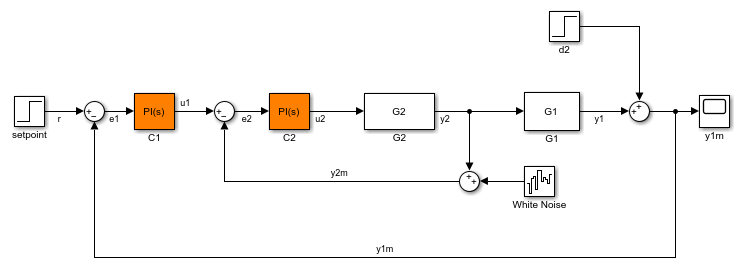Create an slLinearizer interface for the model.

sllin = slLinearizer(mdl); 

Vary the proportional (Kp2) and integral gain (Ki2) of the C2 controller in the 10% range.

Kp2_range = linspace(0.9*Kp2,1.1*Kp2,3); Ki2_range = linspace(0.9*Ki2,1.1*Ki2,5); [Kp2_grid,Ki2_grid] = ndgrid(Kp2_range,Ki2_range); params(1).Name = 'Kp2'; params(1).Value = Kp2_grid; params(2).Name = 'Ki2'; params(2).Value = Ki2_grid; sllin.Parameters = params; 

To calculate the loop transfer function for the inner loop, use the e2 signal as the analysis point. To eliminate the effects of the outer loop, break the outer loop at y1m. Add these points to sllin.

addPoint(sllin,{'e2','y1m'}); 

Determine the index for the maximum values of Ki2 and Kp2.

mdl_index = params(1).Value == max(Kp2_range) & params(2).Value == max(Ki2_range); 

Obtain the inner-loop loop transfer function at e2, with the outer loop open.

sys = getLoopTransfer(sllin,'e2','y1m',-1,mdl_index); 

The fourth input argument specifies negative-feedback for the loop transfer calculation.

mdl = 'watertank'; open_system(mdl)Create a linearization option set, and set the StoreOffsets option.

opt = linearizeOptions('StoreOffsets',true); 

Create slLinearizer interface.

sllin = slLinearizer(mdl,opt); 

Add an analysis point at the tank output port.

addPoint(sllin,'watertank/Water-Tank System'); 

Calculate the loop transfer function at the analysis point, and obtain the corresponding linearization offsets.

[sys,info] = getLoopTransfer(sllin,'watertank/Water-Tank System'); 

View offsets.

info.Offsets 
ans = struct with fields: x: [2x1 double] dx: [2x1 double] u: 1 y: 1 StateName: {2x1 cell} InputName: {'watertank/Water-Tank System'} OutputName: {'watertank/Water-Tank System'} Ts: 0 

## Input Arguments

collapse all

Interface to a Simulink model, specified as either an slLinearizer interface or an slTuner interface.

Analysis point signal name, specified as:

• Character vector or string — Analysis point signal name.

To determine the signal name associated with an analysis point, type s. The software displays the contents of s in the MATLAB® command window, including the analysis point signal names, block names, and port numbers. Suppose that an analysis point does not have a signal name, but only a block name and port number. You can specify pt as the block name. To use a point not in the list of analysis points for s, first add the point using addPoint.

You can specify pt as a uniquely matching portion of the full signal name or block name. Suppose that the full signal name of an analysis point is 'LoadTorque'. You can specify pt as 'Torque' as long as 'Torque' is not a portion of the signal name for any other analysis point of s.

For example, pt = 'y1m'.

• Cell array of character vectors or string array — Specifies multiple analysis point names. For example, pt = {'y1m','y2m'}.

To calculate linsys, the software adds a linearization output, followed by a loop break, and then a linearization input at pt. Consider the following model:Specify pt as 'u'.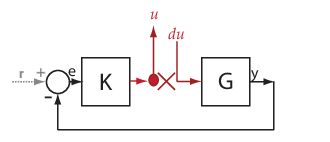The software computes linsys as the transfer function from du to u.

If you specify pt as multiple signals, for example pt = {'u','y'}, the software adds a linearization output, loop break, and a linearization input at each point.du and dy are linearization inputs, and, u and y are linearization outputs. The software computes linsys as a MIMO transfer function with a transfer function from each linearization input to each linearization output.

Feedback sign, specified as one of the following values:

• +1 (default) — getLoopTransfer returns the positive-feedback open-loop transfer function.

• -1getLoopTransfer returns the negative-feedback open-loop transfer function. The negative-feedback transfer function is -1 times the positive-feedback transfer function.

Temporary opening signal name, specified as:

• Character vector or string — Analysis point signal name.

temp_opening must specify an analysis point that is in the list of analysis points for s. To determine the signal name associated with an analysis point, type s. The software displays the contents of s in the MATLAB command window, including the analysis point signal names, block names, and port numbers. Suppose that an analysis point does not have a signal name, but only a block name and port number. You can specify temp_opening as the block name. To use a point not in the list of analysis points for s, first add the point using addPoint.

You can specify temp_opening as a uniquely matching portion of the full signal name or block name. Suppose that the full signal name of an analysis point is 'LoadTorque'. You can specify temp_opening as 'Torque' as long as 'Torque' is not a portion of the signal name for any other analysis point of s.

For example, temp_opening = 'y1m'.

• Cell array of character vectors or string array — Specifies multiple analysis point names. For example, temp_opening = {'y1m','y2m'}.

Index for linearizations of interest, specified as:

• Array of logical values — Logical array index of linearizations of interest. Suppose that you vary two parameters, par1 and par2, and want to extract the linearization for the combination of par1 > 0.5 and par2 <= 5. Use:

params = s.Parameters; mdl_index = params(1).Value>0.5 & params(2).Value <= 5;

The expression params(1).Value>0.5 & params(2).Value<5 uses logical indexing and returns a logical array. This logical array is the same size as params(1).Value and params(2).Value. Each entry contains the logical evaluation of the expression for corresponding entries in params(1).Value and params(2).Value.

• Vector of positive integers — Linear index of linearizations of interest. Suppose that you vary two parameters, par1 and par2, and want to extract the linearization for the combination of par1 > 0.5 and par2 <= 5. Use:

params = s.Parameters; mdl_index = find(params(1).Value>0.5 & params(2).Value <= 5);

The expression params(1).Value>0.5 & params(2).Value<5 returns a logical array. find returns the linear index of every true entry in the logical array

## Output Arguments

collapse all

Point-to-point open-loop transfer function, returned as described in the following:

• If you did not configure s.Parameters and s.OperatingPoints, the software calculates linsys using the default model parameter values. The software uses the model initial conditions as the linearization operating point. linsys is returned as a state-space model.

• If you configured s.Parameters only, the software computes a linearization for each parameter grid point. linsys is returned as a state-space model array of the same size as the parameter grid.

• If you configured s.OperatingPoints only, the software computes a linearization for each specified operating point. linsys is returned as a state-space model array of the same size as s.OperatingPoints.

• If you configured s.Parameters and specified s.OperatingPoints as a single operating point, the software computes a linearization for each parameter grid point. The software uses the specified operating point as the linearization operating point. linsys is returned as a state-space model array of the same size as the parameter grid.

• If you configured s.Parameters and specified s.OperatingPoints as multiple operating point objects, the software computes a linearization for each parameter grid point. The software requires that s.OperatingPoints is the same size as the parameter grid specified by s.Parameters. The software computes each linearization using corresponding operating points and parameter grid points. linsys is returned as a state-space model array of the same size as the parameter grid.

• If you configured s.Parameters and specified s.OperatingPoints as multiple simulation snapshot times, the software simulates and linearizes the model for each snapshot time and parameter grid point combination. Suppose that you specify a parameter grid of size p and N snapshot times. linsys is returned as a state-space model array of size N-by-p.

Linearization information, returned as a structure with the following fields:

Linearization offsets, returned as [] if s.Options.StoreOffsets is false. Otherwise, Offsets is returned as one of the following:

• If linsys is a single state-space model, then Offsets is a structure.

• If linsys is an array of state-space models, then Offsets is a structure array with the same dimensions as linsys.

Each offset structure has the following fields:

FieldDescription
xState offsets used for linearization, returned as a column vector of length nx, where nx is the number of states in linsys.
yOutput offsets used for linearization, returned as a column vector of length ny, where ny is the number of outputs in linsys.
uInput offsets used for linearization, returned as a column vector of length nu, where nu is the number of inputs in linsys.
dxDerivative offsets for continuous time systems or updated state values for discrete-time systems, returned as a column vector of length nx.
StateNameState names, returned as a cell array that contains nx elements that match the names in linsys.StateName.
InputNameInput names, returned as a cell array that contains nu elements that match the names in linsys.InputName.
OutputNameOutput names, returned as a cell array that contains ny elements that match the names in linsys.OutputName.
TsSample time of the linearized system, returned as a scalar that matches the sample time in linsys.Ts. For continuous-time systems, Ts is 0.

If Offsets is a structure array, you can configure an LPV System block using the offsets. To do so, first convert them to the required format using getOffsetsForLPV. For an example, see Approximating Nonlinear Behavior Using an Array of LTI Systems.

Linearization diagnostic information, returned as [] if s.Options.StoreAdvisor is false. Otherwise, Advisor is returned as one of the following:

LinearizationAdvisor objects store linearization diagnostic information for individual linearized blocks. For an example of troubleshooting linearization results using a LinearizationAdvisor object, see Troubleshoot Linearization Results at Command Line.

collapse all

### Loop Transfer Function

The loop transfer function at a point is the point-to-point open-loop transfer function from an additive disturbance at a point to a measurement at the same point.

To compute the loop transfer function at an analysis point, x, the software adds a linearization output, inserts a loop break, and adds a linearization input, dx. The software computes the transfer function from dx to x, which is equal to the loop transfer function at x.

Analysis Point in Simulink ModelHow getLoopTransfer Interprets Analysis Point Loop Transfer Function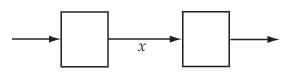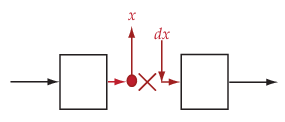Transfer function from dx to x

For example, consider the following model where you compute the loop transfer function at e: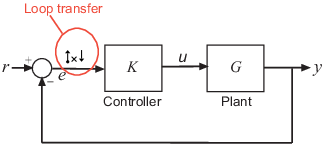Here, at e, the software adds a linearization output, inserts a loop break, and adds a linearization input, de. The loop transfer function at e, L, is the transfer function from de to e. L is calculated as follows:

$e=\underset{L}{\underbrace{-GK}}de.$

To compute -KG, use u as the analysis point for getLoopTransfer.

The software does not modify the Simulink model when it computes the loop transfer function.

### Analysis Points

Analysis points, used by the slLinearizer and slTuner interfaces, identify locations within a model that are relevant for linear analysis and control system tuning. You use analysis points as inputs to the linearization commands, such as getIOTransfer, getLoopTransfer, getSensitivity, and getCompSensitivity. As inputs to the linearization commands, analysis points can specify any open-loop or closed-loop transfer function in a model. You can also use analysis points to specify design requirements when tuning control systems using commands such as systune.

Location refers to a specific block output port within a model or to a bus element in such an output port. For convenience, you can use the name of the signal that originates from this port to refer to an analysis point.

You can add analysis points to an slLinearizer or slTuner interface, s, when you create the interface. For example:

s = slLinearizer('scdcascade',{'u1','y1'});

Alternatively, you can use the addPoint command.

To view all the analysis points of s, type s at the command prompt to display the interface contents. For each analysis point of s, the display includes the block name and port number and the name of the signal that originates at this point. You can also programmatically obtain a list of all the analysis points using getPoints.

For more information about how you can use analysis points, see Mark Signals of Interest for Control System Analysis and Design and Mark Signals of Interest for Batch Linearization.

### Permanent Openings

Permanent openings, used by the slLinearizer and slTuner interfaces, identify locations within a model where the software breaks the signal flow. The software enforces these openings for linearization and tuning. Use permanent openings to isolate a specific model component. Suppose that you have a large-scale model capturing aircraft dynamics and you want to perform linear analysis on the airframe only. You can use permanent openings to exclude all other components of the model. Another example is when you have cascaded loops within your model and you want to analyze a specific loop.

Location refers to a specific block output port within a model. For convenience, you can use the name of the signal that originates from this port to refer to an opening.

You can add permanent openings to an slLinearizer or slTuner interface, s, when you create the interface or by using the addOpening command. To remove a location from the list of permanent openings, use the removeOpening command.

To view all the openings of s, type s at the command prompt to display the interface contents. For each permanent opening of s, the display includes the block name and port number and the name of the signal that originates at this location. You can also programmatically obtain a list of all the permanent loop openings using getOpenings.

#### Introduced in R2013b